﻿ Extension: expression - FHIR v5.0.0-cibuild

This is the Continuous Integration Build of FHIR (will be incorrect/inconsistent at times).
See the Directory of published versions

# Extension: expression

 Vocabulary Work Group Maturity Level: 1 Informative Use Context: Any

URL for this extension:

`http://hl7.org/fhir/StructureDefinition/valueset-expression`

Status: draft. Extension maintained by: HL7

An expression that provides an alternative definition of the content of the value set (compose). There are two different ways to use this expression extension: If both an expression (this extension) and a compose element is present, the compose is understood to the make the same statement as the expression. If there is no compose, the expression is the only definition of the value set, and the value set can only be processed by a server that understands the expression syntax, the expression is computable.

Comment:

The expression may be a reference or the actual expression, and is expected to be in a computable format. Use the rules-text extension for a non-computable expression of the value set definition (compose).

Context of Use: Use on Element ID ValueSet

## Extension Content

Summary

NameFlagsCard.TypeDescription & Constraints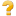expression0..1ExpressionURL = http://hl7.org/fhir/StructureDefinition/valueset-expression
expression: An expression that provides an alternative definition of the content of the value set (compose). There are two different ways to use this expression extension: If both an expression (this extension) and a compose element is present, the compose is understood to the make the same statement as the expression. If there is no compose, the expression is the only definition of the value set, and the value set can only be processed by a server that understands the expression syntax, the expression is computable.

Use on Element ID ValueSetDocumentation for this format

Full Structure

NameFlagsCard.TypeDescription & Constraintsextension0..1ExtensionURL = http://hl7.org/fhir/StructureDefinition/valueset-expression
expression: An expression that provides an alternative definition of the content of the value set (compose). There are two different ways to use this expression extension: If both an expression (this extension) and a compose element is present, the compose is understood to the make the same statement as the expression. If there is no compose, the expression is the only definition of the value set, and the value set can only be processed by a server that understands the expression syntax, the expression is computable.

Use on Element ID ValueSet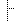extension 0..0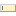url 1..1uri"http://hl7.org/fhir/StructureDefinition/valueset-expression"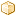value[x] 1..1ExpressionValue of extensionDocumentation for this format

XML Template

```<!-- expression -->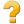<extension xmlns="http://hl7.org/fhir"
url="http://hl7.org/fhir/StructureDefinition/valueset-expression" >
<!-- from Element: extension -->
<valueExpression><!--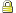1..1 Expression Value of extension --></valueExpression>
</extension>
```

JSON Template

```{ // expression
// from Element: extension
"extension" : [ //  sliced by value:url  in the specified order, Open ]
"url" : "http://hl7.org/fhir/StructureDefinition/valueset-expression", // R!
"valueExpression" : { Expression } // C? R! Value of extension
}
```

Summary

NameFlagsCard.TypeDescription & Constraintsexpression0..1ExpressionURL = http://hl7.org/fhir/StructureDefinition/valueset-expression
expression: An expression that provides an alternative definition of the content of the value set (compose). There are two different ways to use this expression extension: If both an expression (this extension) and a compose element is present, the compose is understood to the make the same statement as the expression. If there is no compose, the expression is the only definition of the value set, and the value set can only be processed by a server that understands the expression syntax, the expression is computable.

Use on Element ID ValueSetDocumentation for this format

Full Structure

NameFlagsCard.TypeDescription & Constraintsextension0..1ExtensionURL = http://hl7.org/fhir/StructureDefinition/valueset-expression
expression: An expression that provides an alternative definition of the content of the value set (compose). There are two different ways to use this expression extension: If both an expression (this extension) and a compose element is present, the compose is understood to the make the same statement as the expression. If there is no compose, the expression is the only definition of the value set, and the value set can only be processed by a server that understands the expression syntax, the expression is computable.

Use on Element ID ValueSetextension 0..0url 1..1uri"http://hl7.org/fhir/StructureDefinition/valueset-expression"value[x] 1..1ExpressionValue of extensionDocumentation for this format

XML Template

```<!-- expression --><extension xmlns="http://hl7.org/fhir"
url="http://hl7.org/fhir/StructureDefinition/valueset-expression" >
<!-- from Element: extension -->
<valueExpression><!--1..1 Expression Value of extension --></valueExpression>
</extension>
```

JSON Template

```{ // expression
// from Element: extension
"extension" : [ //  sliced by value:url  in the specified order, Open ]
"url" : "http://hl7.org/fhir/StructureDefinition/valueset-expression", // R!
"valueExpression" : { Expression } // C? R! Value of extension
}
```

Constraints

• ele-1: All FHIR elements must have a @value or children (xpath: @value|f:*|h:div)
• ext-1: Must have either extensions or value[x], not both (xpath: exists(f:extension)!=exists(f:*[starts-with(local-name(.), 'value')]))
• ele-1: On Extension.extension: All FHIR elements must have a @value or children (xpath on Extension.extension: @value|f:*|h:div)
• ext-1: On Extension.extension: Must have either extensions or value[x], not both (xpath on Extension.extension: exists(f:extension)!=exists(f:*[starts-with(local-name(.), "value")]))
• ele-1: On Extension.value[x]: All FHIR elements must have a @value or children (xpath on Extension.value[x]: @value|f:*|h:div)